# Formula Forensics No. 033 – Interpolation

Recently in my consulting role I came across a not so uncommon question which required me to find the intersection of an unknown Curve with the axis ie: At what date will the project break-even?

Today in Formula Forensics we are going to look at 3 ways to tackle this problem.

## The Problem

The question that was posed was “What is the payback period for the project?

That is at what point (date or time period) is the Cumulative Cash Flow = 0

The cash flow model is summarised below:The pay-back period is defined as the time it takes for the cumulative cash flow to return to zero.

We can see in the table above that it is between 2015 and 2016, and that it will be closer to 2016 than 2015.

Today I will explain 3 techniques for determining what the pay-back period is.

These 3 techniques are:

• Manual Estimation,
• Equal Triangles Solution
• Forecast Formula Solution

## Manual Estimation

Looking at the data table we can see that the Cumulative Cash Flow will return to zero in the later half of 2015.

We can fairly accurately determine this by plotting a chart of the data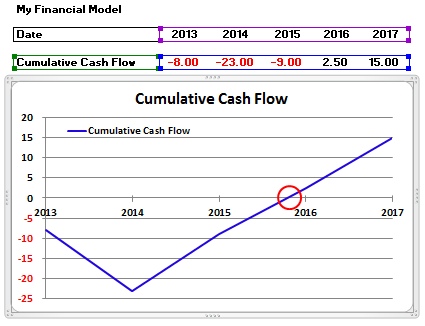We can see here that the line of the Cumulative Cash Flow passes through the X Axis in the October – November period.

We can do a few things to improve our estimate

Firstly add Months as a Minor Unit to the X-AxisNow Zoom in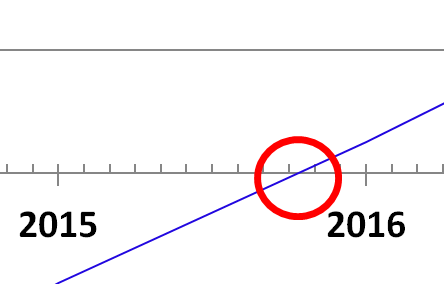We can see that the Cumulative Cash Flow line (Blue) crosses the axis at about the 15th October.

This is 9.5 months into 2015 or 9.5/12 = 0.792 of a year.

Therefor the payback period of the project will be 2.792 Years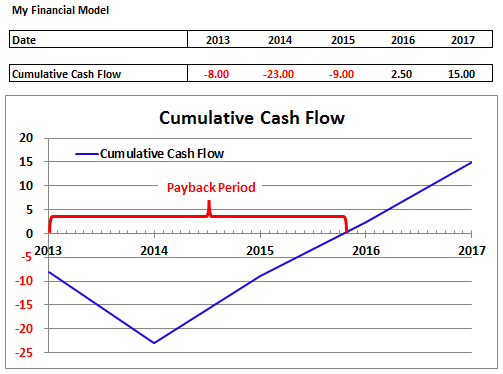## Equal Triangles Solution

The easiest method of determining the exact value is to use the Rule of Triangles, which says the ratio of two sides in two similar triangles is the same.

In our case we can form two triangles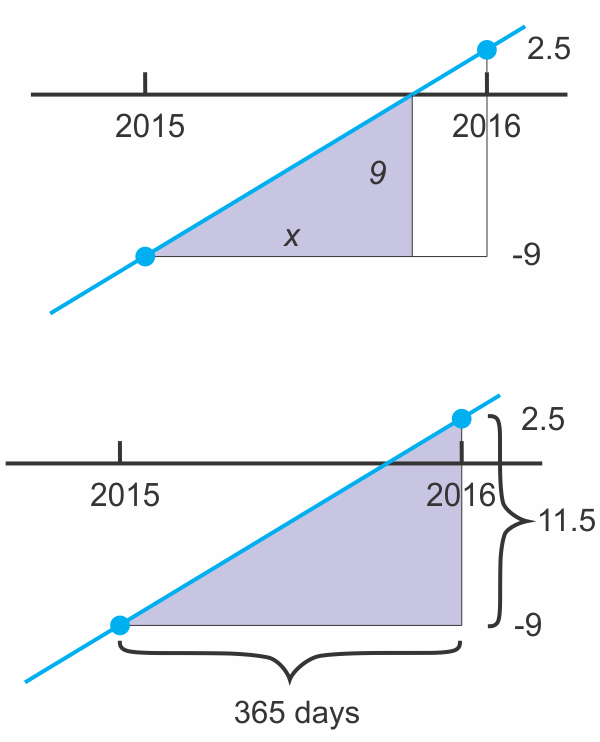Where 9/x = 11.5/365

This can be re-arranged for x as x = (365 x 9)/11.5

x = 285.65 days

or 285.65 = 0.783 Years

Luckily Excel is great at adding dates and days

In a cell type = Date(2015,1,1)+ 285.65

Excel returns 13 Oct 2015

So our pay-back period is 2.78 years

Instead of doing this manually we can write this as a formula in Excel

In a spare cell, say D36 put: =(F4-E4)*(0-E6)/(F6-E6)

Excel displays 285.652 Days

Or as years, in say D37:  =2+(0-E6)/(F6-E6)

Excel displays 2.783 Years

We have to remember that the project started 2 years before 2015 and so the payback is 2.783 years.

## Forecast Formula Solution

Many years ago I stumbled onto a neat web site, http://stackoverflow.com/. The site offers many solutions to various computing problems not just Excel.

Specifically they had answered a similar question about interpolating data points http://stackoverflow.com/questions/1043513/interpolating-data-points-in-excel and specifically the third post which has a formula based solution.

I have used this formula many times in my work roles and here at Chandoo.org Forums and I have seen it used by Faseeh and others as well.

Converting their formula to a Horizontal Range for my cash flow model yields a solution:

=FORECAST(0, OFFSET(C4 , , MATCH(0, C6:G6)-1, 1, 2), OFFSET(C6 , , MATCH(0, C6:G6)-1, 1, 2))

Which we will now pull apart.

The formula =FORECAST(0, OFFSET(C4 , , MATCH(0, C6:G6)-1, 1, 2), OFFSET(C6 , , MATCH(0, C6:G6)-1, 1, 2))

The formula, which appears complex at first, is simply a Forecast() function with other formulas for the Forecast components

=FORECAST(0, OFFSET(C4,,MATCH(0,C6:G6)-1,1,2), OFFSET(C6,,MATCH(0,C6:G6)-1,1,2))

The Excel Forecast() function uses the following syntax: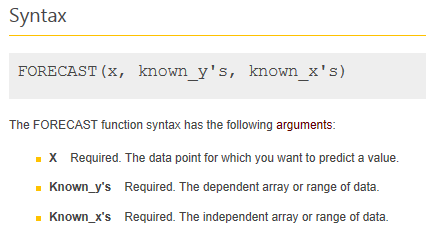In our example

X: 0

Known Y’s: OFFSET(C4,,MATCH(0,C6:G6)-1,1,2)

Known X’s: OFFSET(C6,,MATCH(0,C6:G6)-1,1,2)

You can see directly above that the two Offset formulas in the Known X’s and Known Y’s are exactly the same except that the first refers to the data in Row 4 (The Dates) and the second the data in Row 6 (The Values).

The Forecast() function will return an estimate of Y for a known value of X which is interpolated in the arrays of Known X and Known Y. Forecast uses a least squares method of modelling to form a line of best fit modelling the data.

It is possible to trick Forecast() by not supplying an Array of Data but simply supplying a subset of data which are the points on either side of our known Y value. This is done by using the Offset() function and is described below.

In addition we swap the X and Y values as we are after an unknown X value in our model, knowing Y=0, so by swapping the X and Y values fed into the Forecast() function, Forecast will find the X value which is our required Date.

Going back to the formula:

=FORECAST(0, OFFSET(C4,,MATCH(0,C6:G6)-1,1,2), OFFSET(C6,,MATCH(0,C6:G6)-1,1,2))

We can see that forecast is going to return a Y Value for an X value of 0 where the Known Y’s: are OFFSET(C4,,MATCH(0,C6:G6)-1,1,2) , and Known X’s: OFFSET(C6,,MATCH(0,C6:G6)-1,1,2)

Lets look at the Known Y’s

OFFSET(C4,,MATCH(0,C6:G6)-1,1,2)

This is an Excel Offset() function. Offset() has the syntax:In the example: OFFSET(C6, , MATCH(0,C6:G6)-1, 1, 2)

Reference: C6

Rows: Blank = 0

Columns: MATCH(0,C6:G6)-1

Height: 1

Width: 2

Offset will return a range which is offset from C6 by 0 Rows vertically and MATCH(0,C6:G6)-1 columns and will be 1 Row high and 2 Column Wide.

The section MATCH(0,C6:G6)-1, returns the index number of the location of 0 in the range C6:G6 and subtracts one from that value.

Because Match will not find 0 in the range C6:G6 it will return the location of the next value which is lower than 0 or the position of -9 in our example which is Position 3, subtracting 1 from that to = 2

So our offset formula is now read as

OFFSET(C6, , MATCH(0,C6:G6)-1, 1, 2)

OFFSET(C6, , 2, 1, 2)

We can check this by wrapping the Offset() function in a Sum() function

In cell D48 enter: =SUM(OFFSET(C6,,MATCH(0,C6:G6)-1,1,2))

Or you can enter it as =OFFSET(C6,,2,1,2)

Excel will return -6.5 which is the sum of -9.0 and 2.5

That is explained as Offset cell C6, 0 rows down and 2 Columns to the right (C6 becomes E6) and make the new range 2 cells wide 1 cell high (E6 becomes E6:F6).

We can now see that this returns the two cells that straddle across the Zero position as a Range to the Forecast() function.

Similarilly we can see that the Dates are returned as the Y Values to the Forecast() function by use of the similar:  OFFSET(C4,,MATCH(0,C6:G6)-1,1,2) function  that will convert to E4:F4

Chandoo has written a neat post on how Offset works at: http://chandoo.org/wp/2012/09/17/offset-formula-explained/

Finally we go back to the Forecast() function and see that:

=FORECAST(0, OFFSET(C4,,MATCH(0,C6:G6)-1,1,2), OFFSET(C6,,MATCH(0,C6:G6)-1,1,2))

Is the same as

=FORECAST(0, E4:F4, E6:F6)

In a spare cell, D50 type: =FORECAST(0, E4:F4, E6:F6)

Excel returns 13 Oct 15, our Break-even date.

We can calculate the number of years that this is by subtracting the start date and diving the answer by 365 days:

=(FORECAST(0,OFFSET(C4,,MATCH(0,C6:G6)-1,1,2),OFFSET(C6,,MATCH(0,C6:G6)-1,1,2))-C4)/365

In cell D42, type: =(FORECAST(0,OFFSET(C4,,MATCH(0,C6:G6)-1,1,2),OFFSET(C6,,MATCH(0,C6:G6)-1,1,2))-C4)/365

Excel returns 2.78, which is 2.78 years.

## Other Chandoo.org Posts related to Interpolation

Here at Chandoo.org you can find the following related posts related to Interpolation:

http://chandoo.org/wp/2011/01/24/trendlines-and-forecasting-in-excel/

http://chandoo.org/wp/2011/01/26/trendlines-and-forecasting-in-excel-part-2/

http://chandoo.org/wp/2011/01/27/trendlines-and-forecasting-in-excel-part-3/

Narayank used an Equal Triangles approach to solve: http://chandoo.org/forums/topic/interpolate-a-number-in-between-two-numbers (post 2)

Faseeh then used a Forecast() formula approach as described above, to the same problem (Post 3).

## Final Thoughts

There are many other ways to interpolate values along a series.

I would love to hear about some of your interpolation experiences, techniques or uses

Lets us know in the comments below:

## Formula Forensics “The Series”

This is the 33rd post in the Formula Forensics series.

You can learn more about how to pull Excel Formulas apart in the following posts: Formula Forensic Series

## Formula Forensics Needs Your Help

I need more ideas for future Formula Forensics posts and so I need your help.

If you have a neat formula that you would like to share like above, try putting pen to paper and draft up a Post like above or;

If you have a formula that you would like explained, but don’t want to write a post, send it to Hui or Chandoo.Hello Awesome...

My name is Chandoo. Thanks for dropping by. My mission is to make you awesome in Excel & your work. I live in Wellington, New Zealand. When I am not F9ing my formulas, I cycle, cook or play lego with my kids. Know more about me.

Thank you and see you around.

### Related articles:

 Written by Hui... Tags: Forecast, Interpolate, Interpolation, MATCH(), OFFSET() Home: Chandoo.org Main Page ? Doubt: Ask an Excel Question

### 9 Responses to “Formula Forensics No. 033 – Interpolation”

1.Mitch says:

You can also use intercept instead of forecast. Then you don't need the first 0.

2. [...] Formula Forensics No. 033 – Interpolation [...]

3.RC says:

I adjusted the equal triangle formula to make it automatic and avoid the use of offset in the forecast method.

Assuming X is the last year where the cumulative cashflow was negative, you can find the payback period by the following
=X-1+(0-INDEX(C6:G6,1,X))/(INDEX(C6:G6,1,X+1)-INDEX(C6:G6,1,X))
You can find X by
X =COUNTIF(C6:G6,"<=0")
The result is
=COUNTIF(C6:G6,"<=0")-1+(0-INDEX(C6:G6,1,COUNTIF(C6:G6,"<=0")))/(INDEX(C6:G6,1,COUNTIF(C6:G6,"<=0")+1)-INDEX(C6:G6,1,COUNTIF(C6:G6,"<=0")))

Any way to simplify it? Is it worth avoiding the offset formula for performance purposes?

•Hui says:

@RC
Using a single Offset() function here or there isn't going to affect your workbook's recalculation speed too much, so don't worry about that
It's when people use thousands of then to build tables of data that they seriously degrade performance

4.Hmm says:

[We can see that the Cumulative Cash Flow line (Blue) crosses the axis at about the 15th October. This is 10.5 months into 2015 or 10.5/12 = 0.875 of a year.]
It is wrong.
15th October = 9months + 15days
So, 9.5/12 = 0.79166... of a year.

•Hui... says:

@Hmm
Good pickup
I have corrected the post accordingly

5.Peter says:

Hi,

Years ago searching for an XL approach to non-lineair interpolation i stumbled upon an interesting addin XLXTRFUN (www.xlxtrfun.com)
It is old, and quite some of its function are more or less obsolete.
However, its interpolation function is still very applicable and quite nice - Linear interpolation and more advanced inerpolation like spline and 3D interpolation. This can boost the accuracy if the trends are not really lineair.
Perhaps it is interesting for some of you.

•Hui... says:

@Peter

You may also be interested in a recent Forum post where I wrote a Bi-Linear interpolation formula in a cell not using VBA
http://chandoo.org/forums/topic/plotting-cost-from-a-table-between-known-points

•Peter says:

Hi Hui,
I am still looking for a good example of parabolic or even better cubic interpolation function. I have not yet really managed it well though. Therefore using the XLXtrfun addin.
I would be very interested if you have a solution to that 🙂
Also the example in the post you are referring to would benefit from it in accuracy.

 « Power Pivot & Advanced Excel course is open, Join us today! Distinct Count & Blanks – Power Pivot Real Life Example »

### Get FREE Excel & Power-BI Newsletter

One email per week with Excel and Power BI goodness. Join 100,000+ others and get it free.